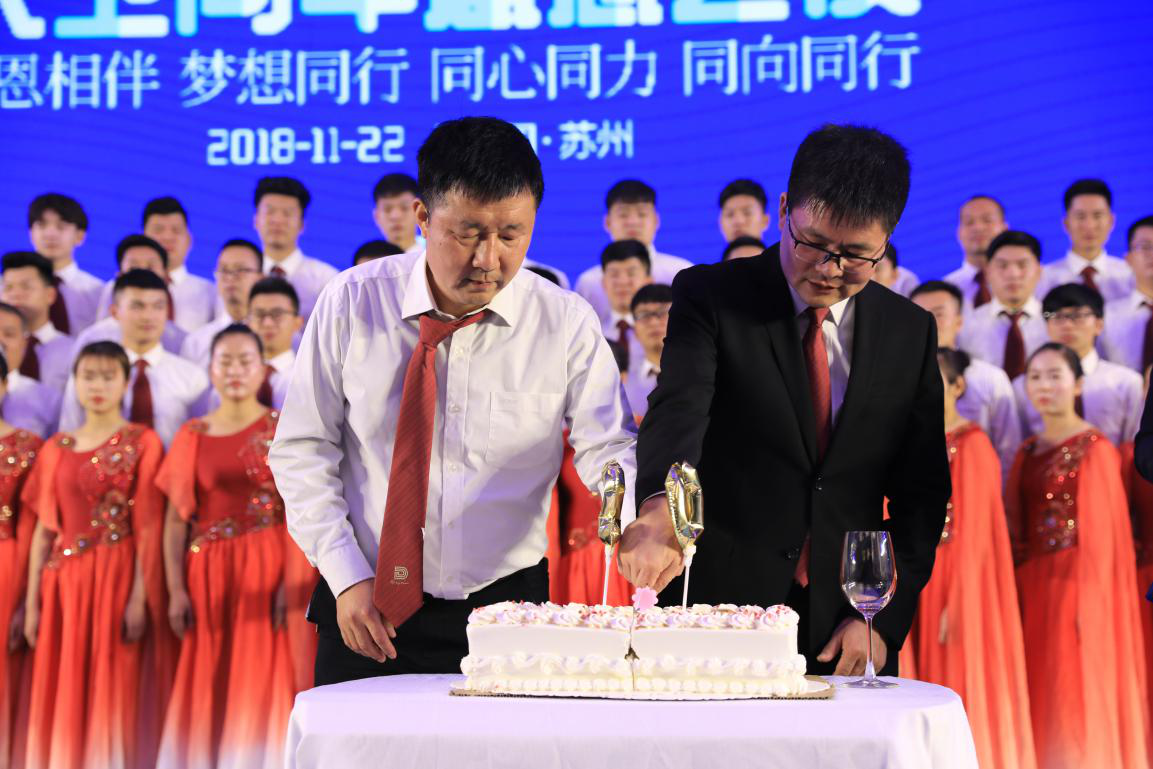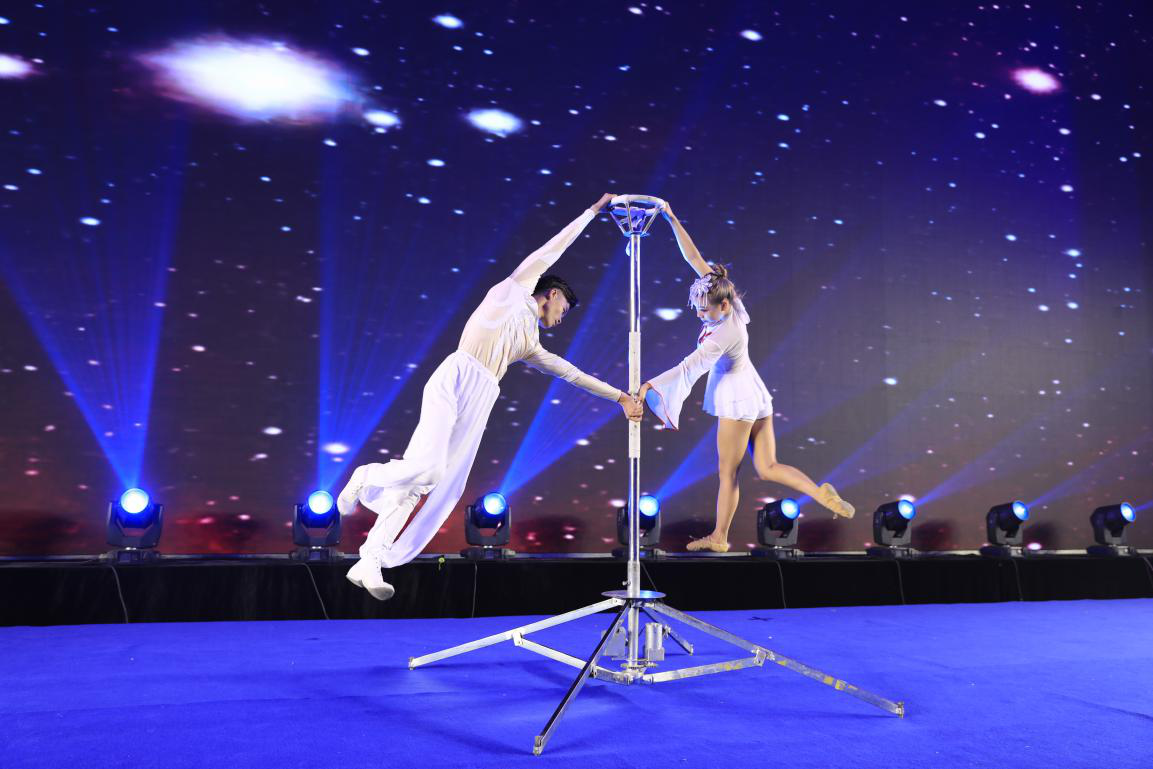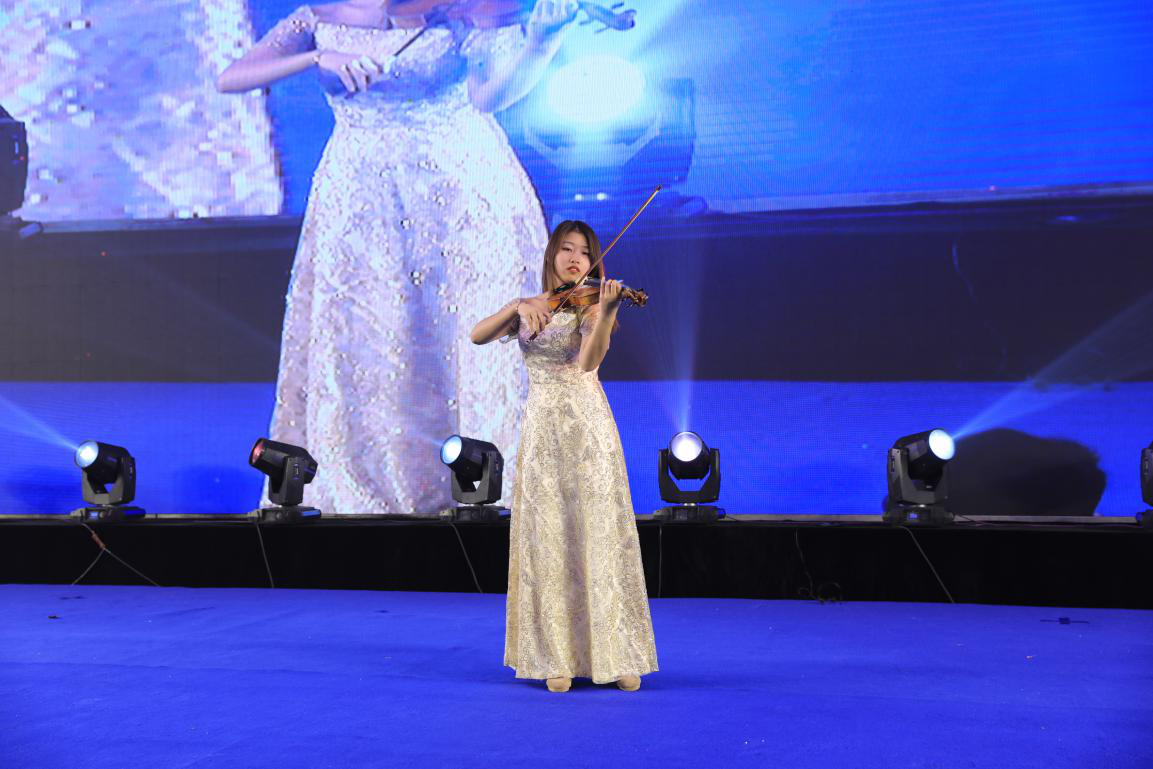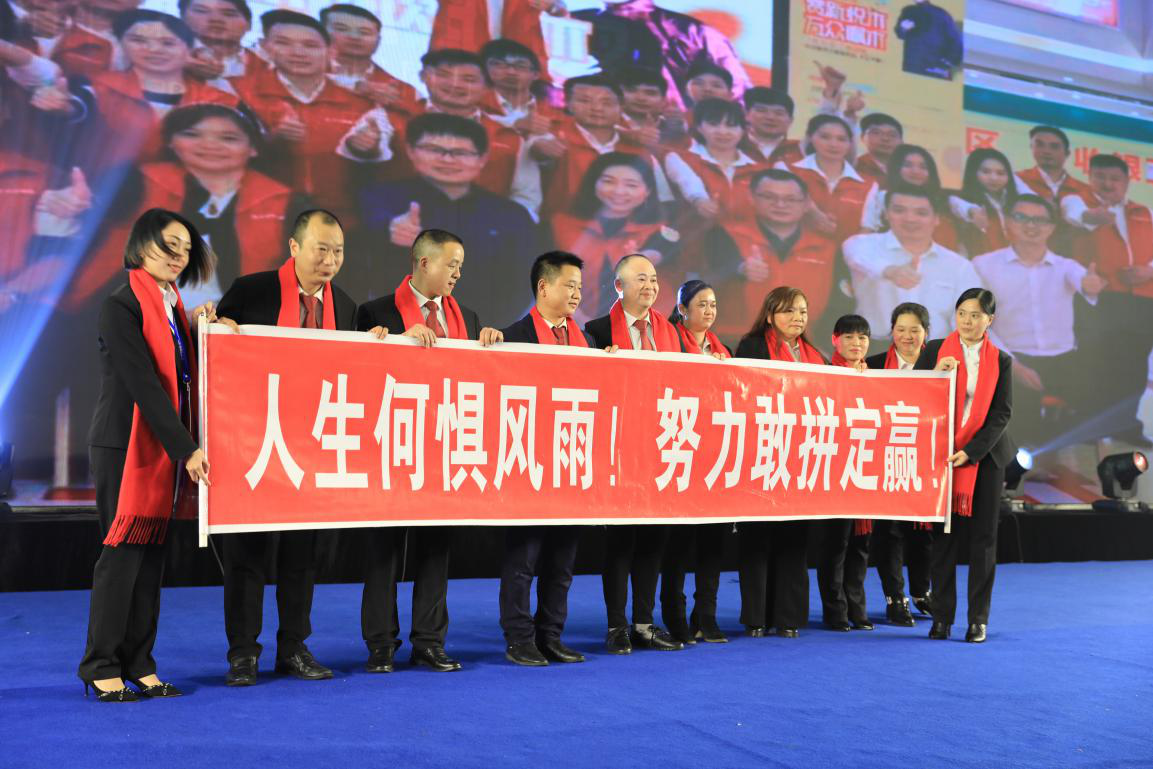|

# 相约大卫 | 献给每一位帮助大卫地板实现梦想的朋友

今夜，感恩之夜！献给每一位帮助大卫地板实现梦想的朋友！今夜，相约大卫！欢聚一堂，开怀畅饮，享受缘分带来的欢乐！璀璨二十载，携手谱华章！20年在人类历史长河中不过是短暂的一瞬，但对大卫地板而言，却是从无到有、从小到大、开天辟地的巨变。大卫地板20年，珍藏的是创业记忆，传承的是精神动力，播洒的是发展希望。抚今追昔，感慨万千、心潮澎湃；展望未来，信心百倍、豪情满怀。站在新的起点上，感恩，振奋，向往。相信在各界领导、朋友、伙伴的一路同行、参与和支持中，大卫地板、XIIF禧梵全屋定制这艘巨轮必将劈波斩浪，稳步前行，为中国林业产业的快速发展打上大卫独有的烙印！晚宴中，大卫地板董事长蒋卫致辞，邀请在场的千人共同举杯，共庆大卫地板二十年华章，共祝大卫地板、XIIF禧梵全屋定制事业未来基业长青。回首过去峥嵘岁月，展望未来锦绣前程，大卫人感恩社会各界的支持；感恩合作伙伴同舟共济，共赴前程；感恩消费者的支持与厚爱。相信自己，选择大卫，志同道合，携手共创，二十年只是一个开始，未来大卫地板、XIIF禧梵全屋定制将创造更多……

相约大卫不负梦想

洒一片暖阳把你我照亮，这支来自各个不同工作岗位员工组成的合唱团，是大卫地板精神的完美诠释。大卫家人们默契的团队配合，节奏铿锵，在一曲气势磅礴、恢弘大气的《相约大卫》中，既唱出了大卫地板发展之歌，又奏响了大卫地板事业未来之路，高潮迭起，欢声不断。将执着专注、协作创新、严谨守序的大卫地板形象演绎的淋漓尽致。如果说这是一群大卫新人，他们身上却有大卫地板独有的匠心印迹；如果说他们是大卫地板老人，这却是一股朝气蓬勃的新鲜力量。带着大卫地板人的匠心精神，他们即将踏上人生的新篇章。正如歌曲所唱“大卫与你相约相望，沉醉千年山水间携手奔跑，不负梦想”！随着工作人员将代表着大卫地板二十周年的纪念蛋糕推上舞台，董事长蒋卫、总经理蒋方，共同上台点燃大卫地板二十周年庆蛋糕蜡烛，共祝大卫地板20周年生日快乐！感恩走过的20年辉煌历程，祝愿大卫地板、XIIF禧梵全屋定制蓄势扬帆再起航！是大卫梦，也是一群人的奋斗梦。从稚气未脱的新人，到事业蒸蒸日上的干将，大卫给予了他们最好的平台。他们在这里成长，他们在这里成功，他们在这里收获自己的梦想。

节目汇演精彩纷呈

此次活动大卫地板精心准备了节目汇演：《江南情》所谓应景，大概就是在这古风古色的江南水乡，看窈窕淑女演绎极具中华艺术神韵之美，言有尽而意无穷的文治“舞”功，不仅让众人眼前一亮，也让大家领略了舞蹈的魅力。《太空漫步》两位演员在道具上攀爬、旋转，柔软而又自然地舒展着躯体，每一个动作都扣人心弦;《现代热舞蹈》更是盖不住的青春活力往外迸发，跟随着音乐仿佛自己也回到了青春时代。器乐演奏大师周红为大家表演了萨克斯独奏《西班牙斗牛士》、《爱在你心口难开》，激烈悠扬的旋律抓住了在场每一位观众的心。古风舞蹈《太湖美》，虽不如之前的表演热烈，但看着她们的舞蹈让人的心情莫名的平静下来，曼妙的舞姿，轻盈的步伐，飘动的衣袖，这是仙女下凡了吧~还有古典雅致的苏州评弹，《花式调酒秀》伴随着音乐，酒瓶在空中翻转，、跳跃，仿佛在随着音乐的节奏翩翩起舞。转眼，就精心调制出一杯杯美酒！携手今宵展望明朝

这是一个友情洋溢的盛会，一个感恩答谢的盛宴。精彩的演出、精致的晚餐、丰富的抽奖环节和精美的礼品，从小米体重秤到华为mate20，满足现场观众们的满满期待，现场共抽取近百名幸运儿，每次抽奖都掀起一个小高潮。活动现场氛围高涨，领导们一一敬酒，拥抱问候我们每一桌的家人，表达20年来对家人们的感激之情。这是一场缘分，希望下一次的盛宴我们还相聚在一起。庆典就此画上圆满的句点，相聚的时间总是短暂，“感恩相伴 梦想同行”大卫地板、XIIF禧梵全屋定制也即将去奋斗更多个二十年！耳边动人的旋律依旧在萦绕着，眼前激昂的步伐也依旧在迈进着。感谢一直支持大卫的您！未来，大卫地板、XIIF禧梵全屋定制将不断创新超越，以平凡创造不平凡，坚守追求与使命，为广大消费者提供更多品质和品位的享受，朝着“百年企业”的愿景，打造享誉世界的家居国际品牌。

相约大卫，献给每一位帮助大卫地板实现梦想的朋友！

`声明：本文由入驻焦点开放平台的作者撰写，除焦点官方账号外，观点仅代表作者本人，不代表焦点立场错误信息举报电话： 400-099-0099，邮箱：jubao@vip.sohu.com，或点此进行意见反馈，或点此进行举报投诉。`A B C D E F G H J K L M N P Q R S T W X Y Z
A - B - C - D - E
• A
• 鞍山
• 安庆
• 安阳
• 安顺
• 安康
• 澳门
• B
• 北京
• 保定
• 包头
• 巴彦淖尔
• 本溪
• 蚌埠
• 亳州
• 滨州
• 北海
• 百色
• 巴中
• 毕节
• 保山
• 宝鸡
• 白银
• 巴州
• C
• 承德
• 沧州
• 长治
• 赤峰
• 朝阳
• 长春
• 常州
• 滁州
• 池州
• 长沙
• 常德
• 郴州
• 潮州
• 崇左
• 重庆
• 成都
• 楚雄
• 昌都
• 慈溪
• 常熟
• D
• 大同
• 大连
• 丹东
• 大庆
• 东营
• 德州
• 东莞
• 德阳
• 达州
• 大理
• 德宏
• 定西
• 儋州
• 东平
• E
• 鄂尔多斯
• 鄂州
• 恩施
F - G - H - I - J
• F
• 抚顺
• 阜新
• 阜阳
• 福州
• 抚州
• 佛山
• 防城港
• G
• 赣州
• 广州
• 桂林
• 贵港
• 广元
• 广安
• 贵阳
• 固原
• H
• 邯郸
• 衡水
• 呼和浩特
• 呼伦贝尔
• 葫芦岛
• 哈尔滨
• 黑河
• 淮安
• 杭州
• 湖州
• 合肥
• 淮南
• 淮北
• 黄山
• 菏泽
• 鹤壁
• 黄石
• 黄冈
• 衡阳
• 怀化
• 惠州
• 河源
• 贺州
• 河池
• 海口
• 红河
• 汉中
• 海东
• 怀来
• I
• J
• 晋中
• 锦州
• 吉林
• 鸡西
• 佳木斯
• 嘉兴
• 金华
• 景德镇
• 九江
• 吉安
• 济南
• 济宁
• 焦作
• 荆门
• 荆州
• 江门
• 揭阳
• 金昌
• 酒泉
• 嘉峪关
K - L - M - N - P
• K
• 开封
• 昆明
• 昆山
• L
• 廊坊
• 临汾
• 辽阳
• 连云港
• 丽水
• 六安
• 龙岩
• 莱芜
• 临沂
• 聊城
• 洛阳
• 漯河
• 娄底
• 柳州
• 来宾
• 泸州
• 乐山
• 六盘水
• 丽江
• 临沧
• 拉萨
• 林芝
• 兰州
• 陇南
• M
• 牡丹江
• 马鞍山
• 茂名
• 梅州
• 绵阳
• 眉山
• N
• 南京
• 南通
• 宁波
• 南平
• 宁德
• 南昌
• 南阳
• 南宁
• 内江
• 南充
• P
• 盘锦
• 莆田
• 平顶山
• 濮阳
• 攀枝花
• 普洱
• 平凉
Q - R - S - T - W
• Q
• 秦皇岛
• 齐齐哈尔
• 衢州
• 泉州
• 青岛
• 清远
• 钦州
• 黔南
• 曲靖
• 庆阳
• R
• 日照
• 日喀则
• S
• 石家庄
• 沈阳
• 双鸭山
• 绥化
• 上海
• 苏州
• 宿迁
• 绍兴
• 宿州
• 三明
• 上饶
• 三门峡
• 商丘
• 十堰
• 随州
• 邵阳
• 韶关
• 深圳
• 汕头
• 汕尾
• 三亚
• 三沙
• 遂宁
• 山南
• 商洛
• 石嘴山
• T
• 天津
• 唐山
• 太原
• 通辽
• 铁岭
• 泰州
• 台州
• 铜陵
• 泰安
• 铜仁
• 铜川
• 天水
• 天门
• W
• 乌海
• 乌兰察布
• 无锡
• 温州
• 芜湖
• 潍坊
• 威海
• 武汉
• 梧州
• 渭南
• 武威
• 吴忠
• 乌鲁木齐
X - Y - Z
• X
• 邢台
• 徐州
• 宣城
• 厦门
• 新乡
• 许昌
• 信阳
• 襄阳
• 孝感
• 咸宁
• 湘潭
• 湘西
• 西双版纳
• 西安
• 咸阳
• 西宁
• 仙桃
• 西昌
• Y
• 运城
• 营口
• 盐城
• 扬州
• 鹰潭
• 宜春
• 烟台
• 宜昌
• 岳阳
• 益阳
• 永州
• 阳江
• 云浮
• 玉林
• 宜宾
• 雅安
• 玉溪
• 延安
• 榆林
• 银川
• Z
• 张家口
• 镇江
• 舟山
• 漳州
• 淄博
• 枣庄
• 郑州
• 周口
• 驻马店
• 株洲
• 张家界
• 珠海
• 湛江
• 肇庆
• 中山
• 自贡
• 资阳
• 遵义
• 昭通
• 张掖
• 中卫

1室1厅1厨1卫1阳台

1
2
3
4
5

0
1
2

1

1

0
1
2
3报名成功，资料已提交审核A B C D E F G H J K L M N P Q R S T W X Y Z
A - B - C - D - E
• A
• 鞍山
• 安庆
• 安阳
• 安顺
• 安康
• 澳门
• B
• 北京
• 保定
• 包头
• 巴彦淖尔
• 本溪
• 蚌埠
• 亳州
• 滨州
• 北海
• 百色
• 巴中
• 毕节
• 保山
• 宝鸡
• 白银
• 巴州
• C
• 承德
• 沧州
• 长治
• 赤峰
• 朝阳
• 长春
• 常州
• 滁州
• 池州
• 长沙
• 常德
• 郴州
• 潮州
• 崇左
• 重庆
• 成都
• 楚雄
• 昌都
• 慈溪
• 常熟
• D
• 大同
• 大连
• 丹东
• 大庆
• 东营
• 德州
• 东莞
• 德阳
• 达州
• 大理
• 德宏
• 定西
• 儋州
• 东平
• E
• 鄂尔多斯
• 鄂州
• 恩施
F - G - H - I - J
• F
• 抚顺
• 阜新
• 阜阳
• 福州
• 抚州
• 佛山
• 防城港
• G
• 赣州
• 广州
• 桂林
• 贵港
• 广元
• 广安
• 贵阳
• 固原
• H
• 邯郸
• 衡水
• 呼和浩特
• 呼伦贝尔
• 葫芦岛
• 哈尔滨
• 黑河
• 淮安
• 杭州
• 湖州
• 合肥
• 淮南
• 淮北
• 黄山
• 菏泽
• 鹤壁
• 黄石
• 黄冈
• 衡阳
• 怀化
• 惠州
• 河源
• 贺州
• 河池
• 海口
• 红河
• 汉中
• 海东
• 怀来
• I
• J
• 晋中
• 锦州
• 吉林
• 鸡西
• 佳木斯
• 嘉兴
• 金华
• 景德镇
• 九江
• 吉安
• 济南
• 济宁
• 焦作
• 荆门
• 荆州
• 江门
• 揭阳
• 金昌
• 酒泉
• 嘉峪关
K - L - M - N - P
• K
• 开封
• 昆明
• 昆山
• L
• 廊坊
• 临汾
• 辽阳
• 连云港
• 丽水
• 六安
• 龙岩
• 莱芜
• 临沂
• 聊城
• 洛阳
• 漯河
• 娄底
• 柳州
• 来宾
• 泸州
• 乐山
• 六盘水
• 丽江
• 临沧
• 拉萨
• 林芝
• 兰州
• 陇南
• M
• 牡丹江
• 马鞍山
• 茂名
• 梅州
• 绵阳
• 眉山
• N
• 南京
• 南通
• 宁波
• 南平
• 宁德
• 南昌
• 南阳
• 南宁
• 内江
• 南充
• P
• 盘锦
• 莆田
• 平顶山
• 濮阳
• 攀枝花
• 普洱
• 平凉
Q - R - S - T - W
• Q
• 秦皇岛
• 齐齐哈尔
• 衢州
• 泉州
• 青岛
• 清远
• 钦州
• 黔南
• 曲靖
• 庆阳
• R
• 日照
• 日喀则
• S
• 石家庄
• 沈阳
• 双鸭山
• 绥化
• 上海
• 苏州
• 宿迁
• 绍兴
• 宿州
• 三明
• 上饶
• 三门峡
• 商丘
• 十堰
• 随州
• 邵阳
• 韶关
• 深圳
• 汕头
• 汕尾
• 三亚
• 三沙
• 遂宁
• 山南
• 商洛
• 石嘴山
• T
• 天津
• 唐山
• 太原
• 通辽
• 铁岭
• 泰州
• 台州
• 铜陵
• 泰安
• 铜仁
• 铜川
• 天水
• 天门
• W
• 乌海
• 乌兰察布
• 无锡
• 温州
• 芜湖
• 潍坊
• 威海
• 武汉
• 梧州
• 渭南
• 武威
• 吴忠
• 乌鲁木齐
X - Y - Z
• X
• 邢台
• 徐州
• 宣城
• 厦门
• 新乡
• 许昌
• 信阳
• 襄阳
• 孝感
• 咸宁
• 湘潭
• 湘西
• 西双版纳
• 西安
• 咸阳
• 西宁
• 仙桃
• 西昌
• Y
• 运城
• 营口
• 盐城
• 扬州
• 鹰潭
• 宜春
• 烟台
• 宜昌
• 岳阳
• 益阳
• 永州
• 阳江
• 云浮
• 玉林
• 宜宾
• 雅安
• 玉溪
• 延安
• 榆林
• 银川
• Z
• 张家口
• 镇江
• 舟山
• 漳州
• 淄博
• 枣庄
• 郑州
• 周口
• 驻马店
• 株洲
• 张家界
• 珠海
• 湛江
• 肇庆
• 中山
• 自贡
• 资阳
• 遵义
• 昭通
• 张掖
• 中卫• 手机• 分享
• 设计
免费设计
• 计算器
装修计算器
• 入驻
合作入驻
• 联系
联系我们
• 置顶
返回顶部